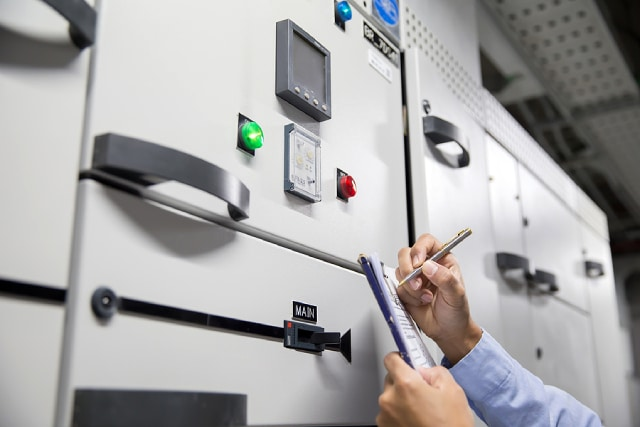Circuits consisting of just one battery and a resistor are very easy to study, but they are not often used in practical applications. We typically see circuits where there are more than two components linked together.

A direct current (DC) electrical circuit is a complete circuit that has current flowing in one directional path. It often consists of a source of DC electricity, such as a battery. They can exist in series, parallel, or a combination of both arrangements.

Voltage, current, and resistance

In a closed electrical circuit, electrical current (measured in amperes, I) is produced by the battery and flows from the negative to positive end. Voltage can refer to either the electrical potential (electromotive force) at a point in the circuit, or the electrical potential difference across two points. Resistance is a measure of the opposition to electrical current flow in a circuit. This is measured in ohms.

Components in a circuit

• Resistor (R)

Resistors are electrical components that provide resistance to a current flow. It is represented in the circuit drawing as a zigzag line.Resistor

• Battery

Batteries are electrical components that supply electrical energy. They have positive and negative terminals. A short line is used to indicate the negative terminal, and the positive terminal is represented with a long line.Battery

• Switch (S)

Switches are used to turn the flow of current in a circuit on and off. When the switch is open, no current flows because there is a gap in the circuit.Switch

Series circuit

In a series circuit, there is only one pathway for current to flow. As an example, you can take a look at the diagram below.

There are 3 resistors (R1, R2, and R3) connected together from the positive terminal of the battery to the negative terminal. As the resistors are connected end-to-end, the current first enters into R1, then the same current enters R2, then R3, and eventually enters into the negative terminal of the battery.

Now, an important thing to remember about series circuits is this: the more components you add, the more work your circuit has to do. So, considering that the total potential difference across the circuit does not change, the voltage across each component will decrease and be split equally. Think about it: How does this affect the brightness of the bulb if you connect 3 bulbs in series, versus just 1 bulb in series?Parallel circuit

In a parallel circuit, all components are connected between the same set of electrically common points. In the diagram below, we also have 3 resistors, but there is more than one path for current to flow. There is a path that goes from 1 to 2 to 7 to 8, and then back to 1 again. There is another path from 1 to 2 to 3 to 6 to 7 to 8, and back to 1 again. The third path is from 1 to 2 to 3 to 4 to 5 to 6 to7 to 8, and back to 1. Each separate path is known as a branch.

The amazing thing about parallel circuits is that, even if you remove one of the resistors from the below example, the circuit can still run, because there are alternative pathways for current to flow. Recall: How would the voltage compare across resistors R1, R2, and R3?Series – parallel circuit

In most real-life applications, electrical circuits are generally a combination of both series and parallel arrangements. In the diagram below, there are two paths for current to flow: first one is from 1 to 2 to 5 to 6 and back to 1 again, and another from 1 to 2 to 3 to 4 to 5 to 6 and back to 1 again. Note how all current pathways go through R1. Therefore in this circuit, R2 and R3 are in parallel arrangement with each other, while R1 is in series arrangement with the parallel combination of R2 and R3.Conclusion

Understanding DC circuits is important for learning about the more complex AC circuits, such as those used in our homes. If you need help on topics such as electrical circuits, you can delve deeper when you attend a H2 Physics Tuition. Here at Best Physics Tuition, we also provide O level physics tuition that will prepare you for the upcoming exams and help you excel.